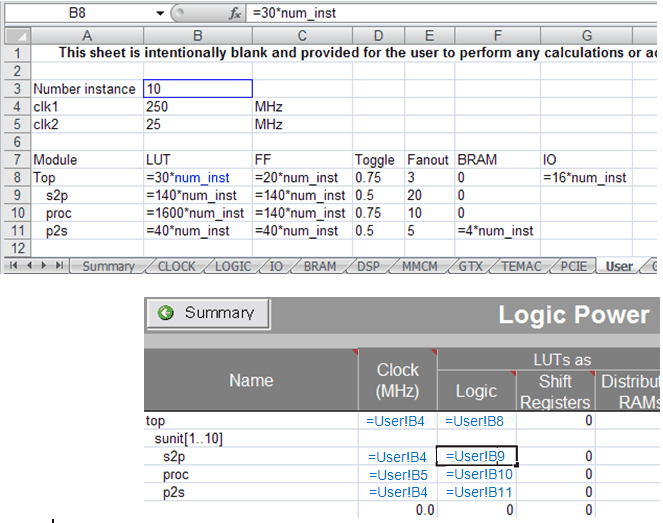# Using Formulas - 2020.2 English

## Xilinx Power Estimator User Guide (UG440)

Document ID
UG440
Release Date
2020-12-04
Version
2020.2 English

With Excel formulas you can simplify data entry, spreadsheet parameterization or create customer reports as explained in the following examples

Example 1
Set clock frequency of all attached synchronous loads in a single place.

Typically a clock net may reach multiple types of resources. Instead of entering the clock frequency on each sheet the following formula can be used on the resource sheets while the clock frequency is only defined once in the Clock sheet. Any change of the clock frequency would immediately be reflected on all the linked resource sheets.

=CLOCK!E19

Example 2
Calculate the fanout sum of all the different loads driven by a clock.

On the clock sheet you might find it useful to enter formulas similar to:

=SUM(LOGIC!G12:I12,BRAM!E10,DSP!E8)

=SUM(IO!I19:K19)

Example 3

Select the GTX data rates to the PCIe interface speed and number of lanes. Entering the following formulae for GTX line rate and number of channels will track the PCIe interface.

• Set channel data rate based on the PCIe bock configuration (if entered on the GTX sheet)

=IF(PCIE!E8="GEN3",8,IF(PCIE!E8="GEN2",5,2.5))

• Set the number of GTX ch0annels to reflect the number of PCIe lanes (if entered on the GTX sheet)

=PCIE!G8

Example 4
Parameterize the spreadsheet entry using formulas and the User sheet. Figure 1 illustrates how to evaluate power when a module is replicated more or fewer times in the design. By varying the number of instances, the quantity of resources for the base blocks, or clock frequency, an Excel formula can automatically recalculate the values which need to be entered in other sheets. In Figure 1, the value for Number instance (named num_inst) in the User sheet automatically calculates utilization and activity for cells that appear in the Logic sheet.
Figure 1. Parameterizing Data Entry Using Formulas on the User Sheet